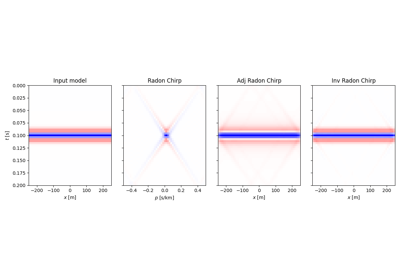Apply Radon forward (and adjoint) transform using Fast Fourier Transform and Chirp functions to a 2-dimensional array of size $$[n_x \times n_t]$$ (both in forward and adjoint mode).

Note that forward and adjoint are swapped compared to the time-space implementation in pylops.signalprocessing.Radon2D and a direct inverse method is also available for this implementation.

Parameters
taxisnp.ndarray

Time axis

haxisnp.ndarray

Spatial axis

pmaxnp.ndarray

Maximum slope defined as $$\tan$$ of maximum stacking angle in $$x$$ direction $$p_\text{max} = \tan(\alpha_{x, \text{max}})$$. If one operates in terms of minimum velocity $$c_0$$, set $$p_{x, \text{max}}=c_0 \,\mathrm{d}y/\mathrm{d}t$$.

dtypestr, optional

Type of elements in input array.

namestr, optional

New in version 2.0.0.

Name of operator (to be used by pylops.utils.describe.describe)

Notes

Refer to  for the theoretical and implementation details.

1

Andersson, F and Robertsson J. “Fast $$\tau-p$$ transforms by chirp modulation”, Geophysics, vol 84, NO.1, pp. A13-A17, 2019.

Attributes
shapetuple

Operator shape

explicitbool

Operator contains a matrix that can be solved explicitly (True) or not (False)

Methods

 __init__(taxis, haxis, pmax[, dtype, name]) Initialize this LinearOperator. adjoint() Hermitian adjoint. apply_columns(cols) Apply subset of columns of operator cond([uselobpcg]) Condition number of linear operator. conj() Complex conjugate operator div(y[, niter, densesolver]) Solve the linear problem $$\mathbf{y}=\mathbf{A}\mathbf{x}$$. dot(x) Matrix-matrix or matrix-vector multiplication. eigs([neigs, symmetric, niter, uselobpcg]) Most significant eigenvalues of linear operator. inverse(x) matmat(X) Matrix-matrix multiplication. matvec(x) Matrix-vector multiplication. reset_count() Reset counters rmatmat(X) Matrix-matrix multiplication. rmatvec(x) Adjoint matrix-vector multiplication. todense([backend]) Return dense matrix. toimag([forw, adj]) Imag operator toreal([forw, adj]) Real operator tosparse() Return sparse matrix. trace([neval, method, backend]) Trace of linear operator. transpose() Transpose this linear operator.

## Examples using pylops.signalprocessing.ChirpRadon2D#